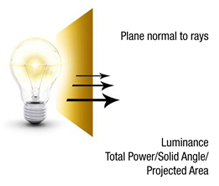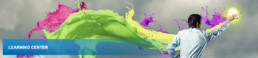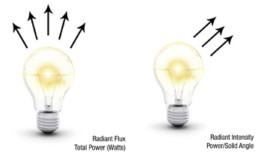Radiometry is the science of the measurement of electromagnetic (EM) radiation. The broader spectrum covered by the science of radiometry is based on physical constants.

The properties of concern to us here are radiated power and its spatial and angular distributions.

The four basic concepts are:

This is the total radiant power emitted from a source or received by a surface. It can also be defined as the rate of flow of radiant energy through a certain area or out of a certain solid angle. The SI unit of radiant flux is the Watt.

It is defined as the directed angular density of radiation from a source. The radiant intensity in a given direction is the sum of the power contained in all the rays (cones) emitted in that direction by the entire source (i.e., power per solid angle). The SI unit for radiant intensity is Watt/Steradian (Watt/sr).

This is a measure of radiant flux incident on an object’s surface (radiant flux per unit area). The SI units for irradiance is Watt/square meter (Watt/m2)

This is a measure of the total radiant intensity per unit projected area. The SI units for radiance is Watt/square meter Steradian (Watt/m2 sr)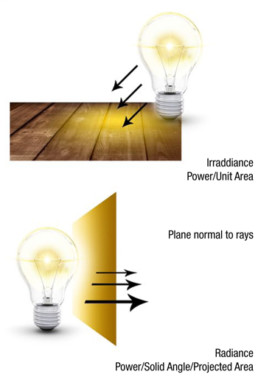Spectroradiometry is the measurement of light energy at individual wavelengths within the electromagnetic spectrum. It can be measured over the entire spectrum or within a specific band of wavelengths.

The radiance of a light source is a single value which is the sum of all energy measured over a spectrum. The individual energy values at a particular wavelength in nanometer (nm) can be determined by a spectral radiance measurement. The SI units for spectral radiance is Watt/square meter Steradian nanometer (Watt/m2 sr nm).

This is a measure of the total radiant intensity per unit projected area. The SI units for spectral irradiance is Watt/square meter nanometer (Watt/m2.nm)

Photometry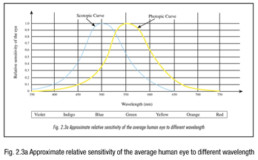Photometry involves measurement of the psychophysical attributes of electromagnetic energy that is visible to the human eye. The use of the term ‘luminous’, which refers to visible light, defines photometry in terms of human perception.

Photometry becomes a modern science in 1942, when Commission Internationale de l’Eclairage (CIE) met to define the response of the average human eye. CIE measured the light-adapted eyes of a sizeable sample group, and compile the data into the CIE Standard Luminosity Function (widely known as photopic curve – chromatic perception at normal state, and scotopic curve – achromatic perception at low level of illuminance. – see Fig.2.3a).

The photometric quantities are related to the corresponding radiometric quantities by the CIE Standard Luminosity Function. We can think of the luminosity function as the transfer function of a filter which approximates the behaviors of the average human eye (Fig. 2.3b).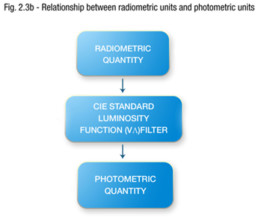Photometry consists of four basic concepts, namely the luminous flux, luminous intensity, illuminance, and luminance.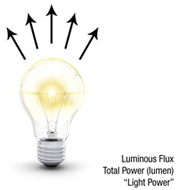Luminous Flux
A source of light radiates energy in the form of electromagnetic waves. We speak of light energy as ‘flux’ and luminous flux is a measure of the flow of light energy emitted by a source, or received by a surface.

The quantity is derived from the radiant flux, W (in Watts), by evaluating the radiation in accordance with the relative luminous efficiency of the ‘standard eye’ (CIE Standard Luminosity Function, V*).

The unit is lumen (lm).

lm = 6 83 x W (Watt) x V*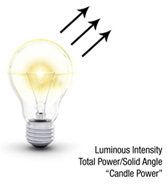Luminous Intensity
This expresses the power of a light source. It is defined as the quantity of luminous flux emitted in a given direction per solid angle (in steradian).

The unit is candela (cd). 1 cd = 1 lumen per steradian. (For practical purposes, one candela power.)

Illuminance
This is a measure of the concentration of luminous flux falling upon a surface.

It is expressed in lumens per unit area.

The unit is lux (lx).
1 lx = 1 lumen per square meter (lm/m2)
The original non-metric British unit is the foot-candle.
1 foot-candle = 1 lumen per square foot (lm/ft2)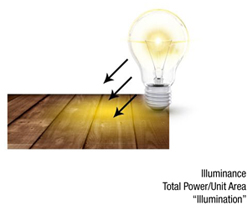Luminance
Also known as photometric brightness, luminance is a measure of the flux emitted from, or reflected by, a relatively flat and uniform surface. Luminance may be thought of as luminous intensity per unit area.

The unit is candelas per square meter (cd/m2), or nit.
The original non-metric British unit is the footlambert (fL)
1 fL = 1 candela/*ft2